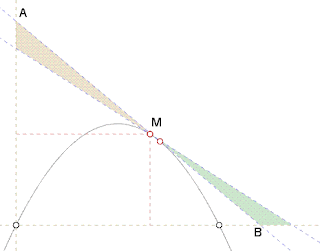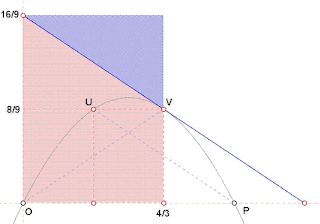## Wednesday, April 28, 2010

### Fox 262 - Solutions

Two solutions to Fox 262
Bleaug:
OAB is minimal for any M fixed (and making AB slope vary) when M is the midpoint of AB. This is intuitively depicted in the following picture where brown and green triangles have the same area (assuming first degree differentiation approximation) which means that OAB area is extremal. This extremum is a minimum because OAB area is a continuous function and it reaches infinity when A or B reaches infinity.This means that the point M we are looking for is such that M is midpoint of AB and AB tangent in M. This brings us to problem 260 where we already encountered point V(4/3, 8/9):
- tangent in V is parallel to UP and vector(UP) = vector(VB) because of parabola property (see Fox 260)
- vector(UP) is symmetric of vector(VO) along y axis because of parabola symmetry
- thus OVB is isoceles and V is the midpoint of ABThus V achieves minimal OAB area.
Area = 4/3 . 16/9 = 64/27.

By Yu:Equation of tangent at x=a, y=-(2a-2)x+a^2.
Area of triangle = (a^4)/(4(a-1)).
When a=4/3, minimum area = 64/27.Question: a=4/3 in Fox 260 and Fox 262. Is this a coincidence?

http://www.8foxes.com/

1.Bleaug's
"This means that the point M we are looking for is such that M is midpoint of AB and AB tangent in M. This brings us to problem 260 where we already encountered point V(4/3, 8/9)"

-binary

2.Bleaug's statement:
"This means that the point M we are looking for is such that M is midpoint of AB and AB tangent in M. This brings us to problem 260 where we already encountered point V(4/3, 8/9)"
3.